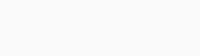# How to Use Standard Deviation to Measure Volatility in Markets

In 2020, the amount of volatility in the market has increased because of Coronavirus and the risks of a recession. As a trader, having a good understanding of volatility can help you make informed decisions.

In the past, we have covered the various ways of identifying volatility, such as using the CBOE volatility index. In this report, we will look at Standard Deviation, another popular tool used to measure volatility in the financial market.

»How to Stay Safe from recessions«

Page Contents

## What is Standard Deviation?

This is a mathematical concept that measures how spread numbers are. In other words, it measures the amount of variation or dispersion in a given set of numbers.

By carefully looking at the dispersion, you can determine whether the asset is in volatility or not. As a result, the Standard Deviation is a good leading indicator you can use to predict the future performance of an asset.

The concept of standard deviation is an important building block for many technical indicators. For example, Bollinger bands are developed by using it. The same is true with the Average True Range indicator, which is used to measure volatility.

### Standard Deviation in Charts

When applied to a chart, the indicator appears as a single line that moves up and down. In most cases, when the price of an asset is trending upwards, the standard deviation is usually relatively low.

However, the indicator tends to rise when there is increased volatility.

## How to calculate it

The process of calculating the standard deviation is relatively long and tough to most people. Still, as we have written before, it is not necessary for you to understand how it is calculated. Instead, you should focus on learning how to interpret it.

1. The first step is to calculate the average or mean price of the financial asset. The period can range from two days to as high as 100 days. Still, the most commonly used period is 14.
2. After identifying the period, you should identify the highest point and subtract it from the mean. By doing this, you have just calculated the period’s deviation.
3. In the next step, you square each period’s deviation and then add the sum of the deviations.
4. The final step is where you divide the sum by the number of observations. After doing this, the deviation will be same to the square root of the number.

### What can affect the deviation

There is an important thing you need to note. The standard deviation will depend on the value of the security. For example a company like Amazon, which is trading at \$1,900, will have a higher deviation than Lending Club, which is trading at less than \$10.

In addition, the resulting deviation will depend on whether the asset experienced a sharp decline or rise in the period under consideration.

The standard deviation is affected by several things. The most important one is the volatility of an asset. A highly volatile asset will have a wider deviation than one with no volatility.

For example, on the chart below, we see that the standard deviation of Bitcoin was substantially lower when it was in a consolidation mode compared to when it was having more volatility.## How to Trade with the Standard Deviation

Unlike other technical indicators,this is not a conclusive one. It will not tell you when or where to enter a buy or sell trade.

Instead, it shows you how volatile an asset is. With this knowledge, you can know whether to hold your trades or exit them. Therefore, standard deviation is better used in combination with other indicators.

### Practical Example

On the chart below, we have added theindicator to a daily chart of Apple. As you can see, during the time when Apple stock was rising, the standard deviation indicator was relatively low.

However, as the Coronavirus illness continued to rise, volatility increased as you can see on the chart below.

### Standard deviation vs variance

Standard deviation is often confused with variance yet the two are incredibly different. While SD is the calculation of how spread numbers are, variance is the average of the squared differences of the mean.

Its calculation uses squares because it weighs outliers more heavily than data close to the mean. While there is no variance indicator in trading, the concept of the standard deviation and variance can be used to predict the future direction of an asset and in portfolio management.

### Summary

The Standard Deviation indicator is different from other indicators because we don’t use it to identify buy and sell opportunities. Instead, it is great in helping you identify when volatility is rising.

Therefore, We recommend that you combine it with other indicators like moving averages and the Fibonacci Retracement tool.

#### External Useful Resources

• Calculating SD for Options Traders - Medium.com
• What Is this concept In Forex? - FXCM

Liked this blog post?

With more than 300,000 subscribers, TraderTV.LIVE™ features a daily live trading broadcast, professional education and an active community.

Accepted Payment Methods:Your ability to open a DTTW trading office or join one of our trading offices is subject to the laws and regulations in force in your jurisdiction. Due to current legal and regulatory requirements, United States citizens or residents are currently unable to open a trading office with us.